# Order type

(diff) ← Older revision | Latest revision (diff) | Newer revision → (diff)

of a totally ordered setA property of the setcharacteristic of every totally ordered setsimilar to. Two setsandthat are totally ordered by relationsandare called similar if there exists a functionthat putsandinto a one-to-one correspondence and is such that for all points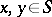one has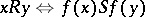. G. Cantor defined the order type as that property of totally ordered sets that remains when the set is considered not with respect to the properties of its elements but with respect to their order. To underline the fact that only this abstraction step takes place, Cantor introduced the symbolto denote the order type of the set. The order types of frequently encountered sets are denoted by special letters. For example, ifis the set of natural numbers ordered by the relation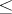, then. If the setof rational numbers is ordered also by the relation, then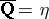. A totally ordered setis of typeif and only if: 1)has a first element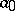; 2) every elementofhas a successor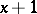; and 3) ifand if the setcontains the successor of each of its elements, then. There exists only one order typeof non-empty sets which are dense, countable and have neither a first nor a last element (Cantor's theorem). A totally ordered set is of type(the type of the set of real numbers) if it is continuous and contains a dense subsetof order type.

One can define operations on order types which are to some extent similar to arithmetical operations.

Letandbe two order types, and letandbe totally ordered sets such that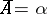,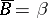and. By the sum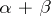one understands the order type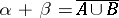, where the set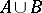is ordered in such a way that all elements ofprecede all elements ofand for bothandthe order is preserved. In particular, ifandare positive integers, then the definition of the sum of order types coincides with the definition of the sum of positive integers. The following equalities are valid:and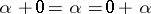, whereis the order type of the empty set. The commutative law does not hold, in general, e.g.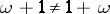.

Let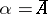and. By the product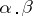one understands the order type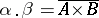, where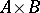is ordered in such a way that if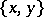,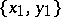are two of its elements, then the first element precedes the second ifor (if the ordinates coincides) if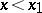(the principle of different last elements). The following equalities hold: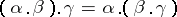,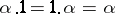,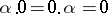, whereis the order type of a one-element set. Multiplication, unlike addition, is not commutative. For instance,. One distributive law holds: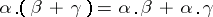. The product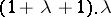represents a continuous order type of the cardinality of the continuum which does not contain a countable dense subset.

Closely related to the sum and the product of order types are the sum of an arbitrary ordered set of order types (see Ordered sum) and the lexicographic product of a well-ordered set of order types. Let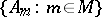be a family of totally ordered sets indexed by a well-ordered set(cf. Well-ordered set), and let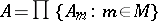be the Cartesian product of this family. The lexicographic product of the familyis the setendowed with the following order. If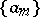andare elements of, thenif and only if either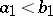, or if there exists ansuch that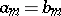for all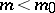and(the principle of different first elements). If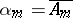andis the lexicographic product of the family, thenis called the product of the family of order types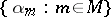. Using the lexicographic product and the generalized continuum hypothesis it is possible to construct for every cardinal numbera totally ordered setof cardinalitysuch that any totally ordered set of cardinality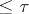is similar to some subset of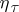. Ifis strongly inaccessible, then the generalized continuum hypothesis is not necessary for the proof of this theorem. In particular, for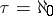(aleph zero) this set may be any totally ordered set of order type.

How to Cite This Entry:
Order type. Encyclopedia of Mathematics. URL: http://encyclopediaofmath.org/index.php?title=Order_type&oldid=11517
This article was adapted from an original article by B.A. Efimov (originator), which appeared in Encyclopedia of Mathematics - ISBN 1402006098. See original article